# Test: Patterns - 2

## 10 Questions MCQ Test Mathematics for Class 1 | Test: Patterns - 2

Description
Attempt Test: Patterns - 2 | 10 questions in 15 minutes | Mock test for Class 1 preparation | Free important questions MCQ to study Mathematics for Class 1 for Class 1 Exam | Download free PDF with solutions
QUESTION: 1

### Choose the correct option that completes the given pattern.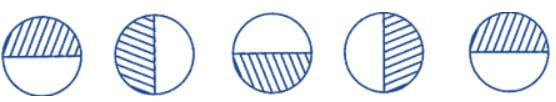Solution:

The pattern is moving anti-clockwise.

QUESTION: 2

### What comes next in the pattern? AABBCCDDAB ______ DAAABBBCCCDDD

Solution:

As the alphabets are repeating twice, then once and then thrice.

QUESTION: 3

### Complete the number pattern.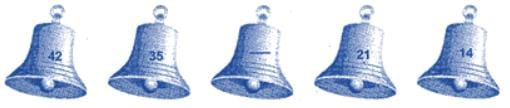Solution:

The difference between numbers is 7 in decreasing order.

QUESTION: 4

Study the number patterns. Find the missing numbers in empty row.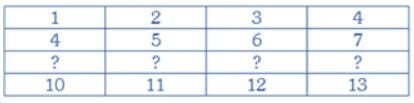Solution:

The difference between numbers of horizontal row is 1 and last number is repeating in next row.

QUESTION: 5

What will be the next to complete the following pattern?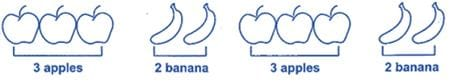Solution:

Pattern of 3 apples, and 2 banana is repeating so, next pattern, will be of three apples.

QUESTION: 6

What comes next in the pattern?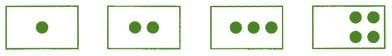Solution:

The difference between the numbers of dots is 1. So, next box will have 5 dots.

QUESTION: 7

What comes in the blank circle?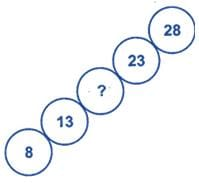Solution:

The difference between numbers is 5. Use skip counting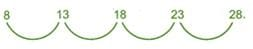QUESTION: 8

Find the missing number.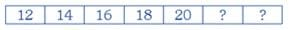Solution:

The difference between numbers is 2.

QUESTION: 9

Which one of the following options is correct to complete the numbers in the ladder?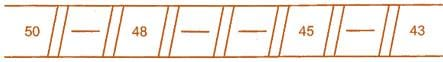Solution:

The difference between numbers is 1.

QUESTION: 10

Study the numbers in the diagrams given below. Identify each pattern. Fill in the missing number in the empty row.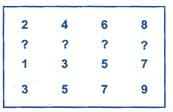Solution:

There is difference of 2 between each number of horizontal row.Use Code STAYHOME200 and get INR 200 additional OFF Use Coupon Code